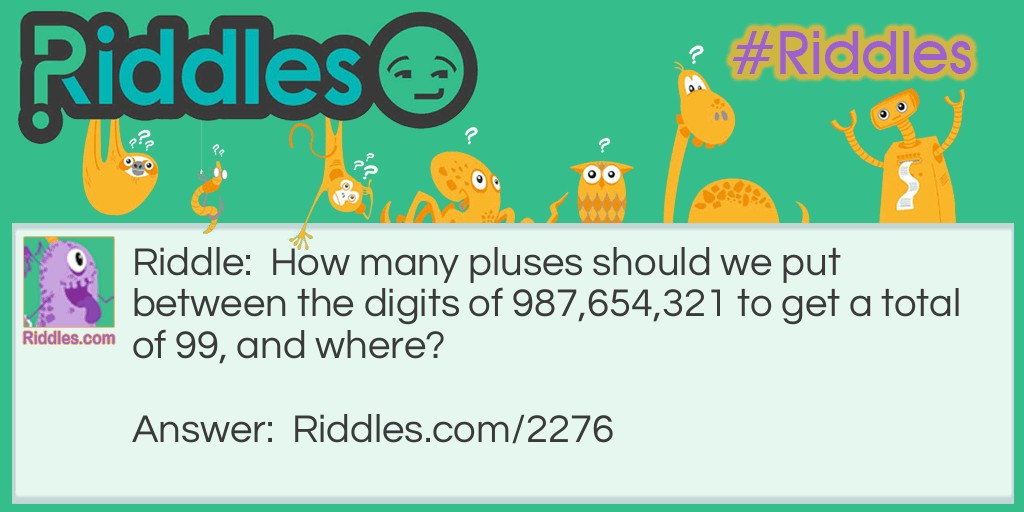ADVERTISEMENT
Riddle:

How many pluses should we put between the digits of 987,654,321 to get a total of 99, and where?

Answer:

9 + 8 + 7 + 65 + 4 + 3 + 2 + 1= 99

9 + 8 + 7 + 6 + 5 + 43 + 21= 99

VOTE
Rated 3/5 based on 618 votes
SHARE
COMMENTAdd Plus Signs Riddle Meme with riddle and answer page link.
ADVERTISEMENT